Next: Graphical representation of phonons Up: Description of the program Previous: Partial density of states

## Translational invariance

When all the atoms are moved by the same amount, i.e. the crystal is rigidly shifted, the force on each atom must be zero. This is a stronger constraint than the one in which it is the sum of the forces on each atom to be zero. The latter is expressed by: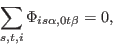(9)

where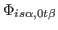is the force constant matrix,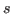and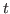run over the number of atoms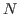in the primitive cell and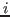over the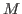lattice vectors included in the calculation. If this constraint is not satisfied, it is straightforward to impose it by subtracting from the calculated force on each atom the value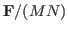, where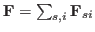, and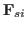is the force acting on atomin primitive cell.

The former condition is: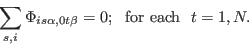(10)

Clearly, Eq. 10 implies Eq. 9, but the opposite is not true in general. However, it is Eq. 10 to imply that at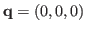the three acoustic branches have identically zero frequencies.

The constraint in Eq. 10 has to be imposed in such a way that the force constant matrix remains symmetric: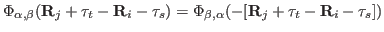. In the PHON code this is done iteratively, in a number of steps in which the symmetry is re-imposed at each step.

To impose translational invariance as described set the variable:

NTI = 20

Translational invariance is imposed iteratively, and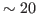iterations are usually enough.

The amount of output printed by the PHON is controlled by the variable IPRINT. IPRINT=0 will produce a minimal output, IPRINT=3 a verbose output, which also includes the dynamical matrix and its eigenvectors.Next: Graphical representation of phonons Up: Description of the program Previous: Partial density of states
Dario Alfe` 2012-02-20